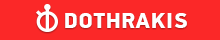GMAT Question of the Day - Daily to your Mailbox; hard ones only

 It is currently 26 Jun 2019, 03:57GMAT Club Daily Prep

Thank you for using the timer - this advanced tool can estimate your performance and suggest more practice questions. We have subscribed you to Daily Prep Questions via email.

Customized
for You

we will pick new questions that match your level based on your Timer History

Track

every week, we’ll send you an estimated GMAT score based on your performance

Practice
Pays

we will pick new questions that match your level based on your Timer History

Not interested in getting valuable practice questions and articles delivered to your email? No problem, unsubscribe here.Show Tags00:00

Difficulty:25% (medium)

Question Stats:95% (01:29) correct5% (01:19) wrongbased on 32 sessions

HideShow timer StatisticsThe total charge for a 4-week dance class is $55.00. The class has 3 sessions per week, and each session lasts 45 minutes. Approximately what is the charge per hour for the class? A.$18.30
B. $13.75 C.$6.10
D. $5.35 E.$4.60

_________________
InternB
Joined: 17 Apr 2018
Posts: 9
Location: Thailand
Concentration: Finance, Economics
GMAT 1: 610 Q46 V28GMAT 2: 720 Q50 V38Show Tags

Total number of minutes = $$45$$(minutes per session)*$$3$$(times a week)*$$4$$(no.of weeks) = 540
Total number of hours $$= \frac{540}{60} = 9$$ hours
Per hour charge $$= \frac{55}{90} = 6.1$$

Hence C, $$6.1$$
_________________
Regards,

Vaibhav

Sky is the limit. 800 is the limit.

~GMAC
InternJoined: 24 Nov 2018
Posts: 6
Re: The total charge for a 4-week dance class is $55.00. The class has 3 [#permalink] Show Tags My Solution:$55= number of classes
$55= 4(3)$55=12
12 classes =12 hours

BUT 12(.75)=9 hours since a class only last 3/4 of an hour
55/9= $6.1 per class CEOP Joined: 18 Aug 2017 Posts: 3937 Location: India Concentration: Sustainability, Marketing GPA: 4 WE: Marketing (Energy and Utilities) Re: The total charge for a 4-week dance class is$55.00. The class has 3  [#permalink]

Show Tags

1
Bunuel wrote:
The total charge for a 4-week dance class is $55.00. The class has 3 sessions per week, and each session lasts 45 minutes. Approximately what is the charge per hour for the class? A.$18.30
B. $13.75 C.$6.10
D. $5.35 E.$4.60

using the information we can say :

(55*60/( 4*3*45)
= $6.10 option C _________________ If you liked my solution then please give Kudos. Kudos encourage active discussions.Re: The total charge for a 4-week dance class is$55.00. The class has 3   [#permalink] 26 Nov 2018, 21:59
Display posts from previous: Sort by

The total charge for a 4-week dance class is \$55.00. The class has 3